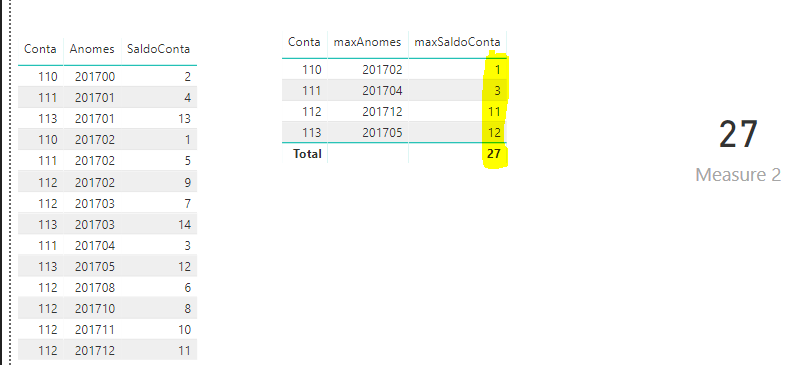cancel
Showing results for
Did you mean:Impactful Individual

## Sum the value of one column based on the maximum value of another column

I need a measure to add the last non blank value from column SaldoConta from the table pcsabelow from each column conta.

The criteria to define last non blank is the one with maximum value in column AnoMes.I've tried a million different DAX expressions but it seems impossible to get what I want.

It should add only the LAST nonblank value in AnoMes, so for conta 110, it should be 112,56.

1 ACCEPTED SOLUTIONResident Rockstar

Hi @webportal,

You could create a formula below to group the max Anomes and Conta.

```t =
SUMMARIZE ( 'table', [Conta], "maxAnomes", MAX ( 'table'[Anomes] ) ),
"maxSaldoConta", LOOKUPVALUE (
'table'[SaldoConta],
'table'[Conta], [Conta],
[Anomes], [maxAnomes]
)
)```

Then you could create the measure to calculate the sum of [maxSaldoConta].

Here is the output.Best  Regards,

Cherry

Community Support Team _ Cherry Gao
If this post helps, then please consider Accept it as the solution to help the other members find it more quickly.
3 REPLIES 3Resident Rockstar

Hi @webportal,

You could have a try with the formula below.

```Measure =
IF (
LASTNONBLANK ( 'Table'[Anomes], 1 ),
CALCULATE (
MAX ( 'Table'[SaldoConta] ),
FILTER (
ALL ( 'Table' ),
'Table'[Conta] = MAX ( 'Table'[Conta] )
&& 'Table'[Anomes] = MAX ( 'Table'[Anomes] )
)
)
)
```

Here is the output.If I miss something, please share your desired output, so that we can help further investigate on it?

Best Regards,

Cherry

Community Support Team _ Cherry Gao
If this post helps, then please consider Accept it as the solution to help the other members find it more quickly.Impactful Individual

Hi @v-piga-msft thank you for helping!

The issue here is I don't have a filter context for "conta" and I need to sum the values of "SaldoConta" of the rows that correspond to the maximum "AnoMes" of each 'conta' - which can be different for each "conta".Resident Rockstar

Hi @webportal,

You could create a formula below to group the max Anomes and Conta.

```t =
SUMMARIZE ( 'table', [Conta], "maxAnomes", MAX ( 'table'[Anomes] ) ),
"maxSaldoConta", LOOKUPVALUE (
'table'[SaldoConta],
'table'[Conta], [Conta],
[Anomes], [maxAnomes]
)
)```

Then you could create the measure to calculate the sum of [maxSaldoConta].

Here is the output.Best  Regards,

Cherry

Community Support Team _ Cherry Gao
If this post helps, then please consider Accept it as the solution to help the other members find it more quickly.